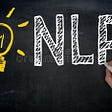# How to use the Learning Rate Finder in TensorFlow

When working with neural networks, every data scientist must make an important choice: the learning rate. A bad learning rate can stop your network from successfully training. In this article, I’m going to teach you a simple, robust way to find a good learning rate for your neural networks.

Why does the learning rate matter so much? Let’s look at a few different scenarios. First, consider a minimal learning rate, much smaller than the ideal learning rate. Each iteration of training, the network updates its weights using the below formula:

Where θ is the set of the weights that a network learns which successfully fit the data. The ⍺ refers to the learning rate which controls the update of the network weights. J(θ) is called the loss function. A loss function helps to measure the correctness of the model in terms of its ability to estimate the relationship between X (input data) and y (output label).

As you can see in the above formula, if alpha is really small, then the network won’t update its weights by much at all. So in that scenario, the network takes a longer time to find the set of weights that successfully fit the data.

On the other hand, if alpha is really large, then the network updates its weights in large increments. So in that scenario, we overshoot the ideal weights, and worse, may continue to miss the optimum weights forever as our optimizer ricochets around.

Therefore, a very large learning rate results in loss divergence, i.e. loss increases very fast, and we never reach the minimum, whereas a very low learning rate results in loss plateau at first and takes a long time to reach the minimum. Between these two extremes, there exists a good learning rate. Thus, the learning rate is a crucial hyper-parameter.

We can use the learning rate finder (LRFinder) to find a good learning rate.

# What is LRFinder

The idea of the learning rate finder (LRFinder) comes from a paper called “Cyclical Learning Rates for Training Neural Networks” by Leslie Smith. The learning rate finder is a method to discover a good learning rate for most gradient based optimizers.

The LRFinder method can be applied on top of every variant of the stochastic gradient descent¹, and most types of networks. However, some complex network setups, e.g. GANs, may not work with the LRFinder (at least not without some thought and research).

# How to use the LRFinder

Given an untrained neural network, a loss function and training data, take the following steps:

1. Start with a very small learning rate (e.g. 1e-10) and exponentially increase the learning rate with each training step. Here’s how to do this in the model function of your network:
`# decay_learning_rate = learning_rate *                        decay_rate ^ (global_step / decay_steps)learning_rate = tf.train.exponential_decay(1e-10, global_step=global_step, decay_steps=your_value, decay_rate=your_value)# So it can be seen in TensorBoard latertf.summary.scalar('learning_rate', learning_rate)`

You need to manually set the decay_steps and decay_rate based on your network. To see this code in situ, have a look at our example.

2. Train your network as normal.

3. Record the training loss and continue until you see the training loss grow rapidly.

4. Use TensorBoard to visualize your TensorFlow training session. Analyse the loss to determine a good learning rate (I’ll explain how in the next section).

## How to determine a good learning rate

You can identify a learning rate by looking at the TensorBoard graph of loss against training step. You want find the section where loss is decreasing fastest, and use the learning rate that was being used at that training step.

Here are the TensorBoard graphs from a real world example:

We are able to identify the regions of loss plateau, reducing loss and loss explosion in the above loss graph as shown below. Loss plateau refers to the region where the learning rate is too small for the network to make any progress at minimizing its loss:

A good estimate of a learning rate is the one being used during the fastest rate of loss decrease. In the below graph, the steepest part lies roughly at training step 4.4k.

Using the graph of learning rate against training step, we can find out what learning rate was being used at step 4.4k. It was 1.03e-5 . This is the learning rate we’ll use for our network.

This method is an approximation. It works fairly reliably in practice. Here are some of the assumptions that it relies upon:

1. A good learning rate generally performs well across all training steps (e.g. if we encounter a learning rate that performs well at a particular training step, then we can use it for all of training).
2. We increased the learning rate fast enough that a good one was tried before the network found its parameters converged on a loss minima (if it got that far before the learning rate increased enough to cause divergence)

# LRFinder vs. Grid search

Grid search involves full training with different values of the learning rate. Then, we choose the learning rate which had the lowest final loss as a good learning rate. Training the network many times, to try each different learning rate takes a lot of time and resources. In the case of LRFinder, we only train the network once. Therefore LRFinder requires much less time and resources than grid search. However, grid search is robust and exhaustive in a way LRFinder cannot be. For more complex networks, or hard to optimize hyper-parameters, grid search may be a necessary tool.

To better understand the affect of optimizer and learning rate choice, check this article by David Mack. The article shows that the right hyper-parameters are crucial to training success, yet can be hard to find.

Octavian’s mission is to develop systems with human-level reasoning capabilities. We believe that graph data and deep learning are key ingredients in making this possible. If you are interested in learning more about our research or contributing, check out our website or get in touch.

## Acknowledgements

I would like to thank David Mack for coming up with the idea of writing an article about LRFinder, also helping in reviewing and editing the article. I would like to thank Andrew Jefferson for his input on the learning rate finder method implementation in TensorFlow.

--

--

--

## More from Octavian

Research into machine learning and reasoning

## [PR113] D8: StyleGAN2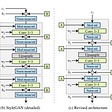## Brain Tumor Segmentation of MRI images with CNN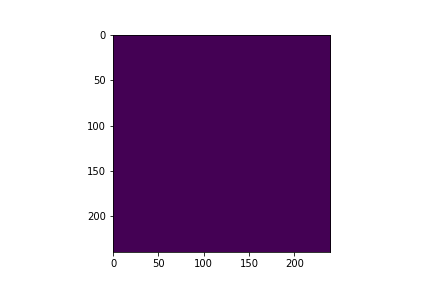## Cartpole - Introduction to Reinforcement Learning (DQN - Deep Q-Learning)## MUSICVAE — Understanding of the google’s work for interpolating two music sequences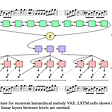## Advancing over BERT ? BigBird, ConvBERT, DynaBERT ...## What are Type I and Type II errors? Which one is more risky/dangerous? How can it be reduced?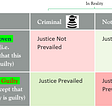## Image Convolution From Scratch## Intro to CASP for Machine Learning Researchers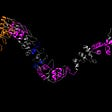## Ashwath Salimath

Data Scientist at SSE Airtricity | Octavian.AI | Omdena

## Sequence to Sequence models (with implementation — make a translator) — Part 2## How To Teach Machines To Learn Like Humans | Dataloop Blog## Generating Alt Text For Memes Using Machine Learning## Stemming & Lemmatization